### Home > PC > Chapter 1 > Lesson 1.1.3 > Problem1-40

1-40.
1. Find a reasonable equation for each function graphed below. Homework Help ✎

1.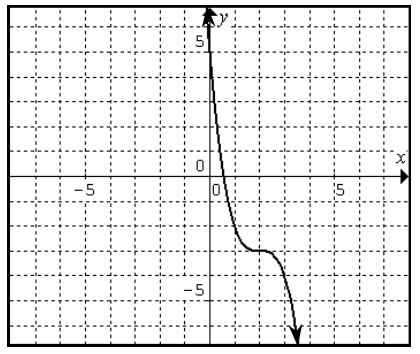2.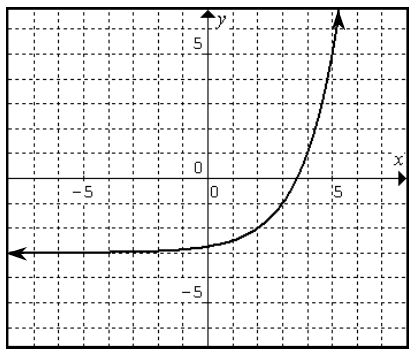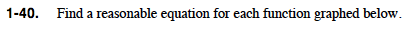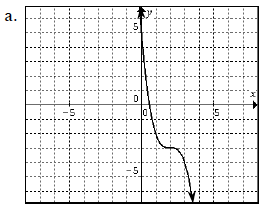What parent function resembles this curve?

Where is the locator point?

What is the general equation of a cubic function?

Substitute the locator point for the horizontal and vertical shifts.

How should the equation show that the curve is reflected vertically?

A cubic function is a possible answer.

(2, −3)

y = a(xh)3 + k

y = a(x − 2)3 − 3

y = −(x − 2)3 − 3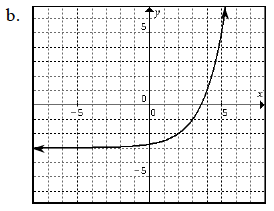Follow a similar procedure as outlined in part (a).